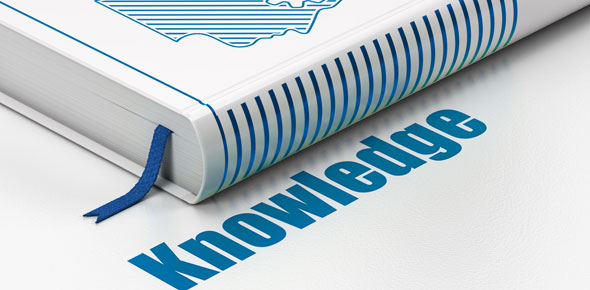# Aseptic II Practice Exame

83 Questions | Total Attempts: 51SettingsPractice for Uyen's exam on 11-4-09

• 1.
Most IV medications are given how long, after preparation to expire?
• A.

6 hours

• B.

24 hours

• C.

2 days

• D.

1 week

• E.

None of the above

• 2.
What is the angle for hand positioning when working in a BSC?
• A.

30 degrees

• B.

45 degrees

• C.

90 degrees

• D.

180 degrees

• E.

None of the above

• 3.
What is another brand name for Kefzol?
• A.

Keflex

• B.

Ancef

• C.

Flagyl

• D.

Fortaz

• E.

None of the above

• 4.
When making a TPN, we usually add Lipids (if we add lipids)
• A.

In the beginning

• B.

In the middle

• C.

At the end

• D.

It does not matter when we add it

• E.

None of the above

• 5.
The concentration of a stock drug is 500 mg/14mL, you need to make 225 mg in D5W 100mL, how much drug will you draw up in your syringe?
• A.

2.25 mL

• B.

6.2 mL

• C.

63 mL

• D.

6 mL

• E.

6.3 mL

• 6.
How much D70W do you need when you need to make 1L of D5W and your other available stock is water?
• A.

80 mL

• B.

929 mL

• C.

0.71L

• D.

0.17L

• E.

None of the above

• 7.
How much D5W do you need when you need to make 750mL of D15W and your other available stock D5W?
• A.

0.167L

• B.

0.833L

• C.

83 mL

• D.

170 mL

• E.

None of the above

• 8.
What is our Room Number?
• A.

IB1421

• B.

CC1421

• C.

TB1421

• D.

ED1841A

• E.

None of the above

• 9.
When working in an LAFH, you need work within _______ inside hood
• A.

3 inches

• B.

4 inches

• C.

6 inches

• D.

1 foot

• E.

None of the above

• 10.
What is the abbreviation for tatol parenteral nutrition?
• A.

PPN

• B.

HA

• C.

TPN

• D.

TIVN

• E.

None of the above

• 11.
What is thed for imipenem/cilastatin?
• A.

Timentin

• B.

Zosyn

• C.

Primaxin

• D.

Unasyn

• E.

None of the above

• 12.
When taking the Chemo Preparation Test, you are allowed, how many spots?
• A.

1 dime size

• B.

2 nickel size

• C.

2 dime size

• D.

All of the Above

• E.

None of the above

• 13.
What is the generic for Demadex?
• A.

Hydromorphone

• B.

Torsemide

• C.

Dexamethasone

• D.

Vasopressin

• E.

None of the above

• 14.
What is the generic for Gamunex?
• A.

IVIG

• B.

Gamminmmune

• C.

Garamycin

• D.

Carimune

• E.

None of the above

• 15.
BSA stands for
• A.

Basal surface area

• B.

Body, Size, Area

• C.

Body surface area

• D.

All of the above

• E.

None of the above

• 16.
What is the equation for calculating Factor A?
• A.

(Infuse Volume/Total Volume) X Wt

• B.

(Total Volume/Infuse Volume) X Wt

• C.

(Total Volume/(T-M-F X24hr)) X Wt

• D.

A & B

• E.

A & C

• 17.
To prepare sterile products, the LAFH should be running for a minimum of _____ before use.
• A.

30 seconds

• B.

60 minutes

• C.

30 minutes

• D.

1 day

• E.

None of the above

• 18.
What does PRN stand for?
• A.

As necessary

• B.

As needed

• C.

When needed

• D.

All of the above

• E.

None of the above

• 19.
Needles used to prepare IV solutions should be disposed in?
• A.

Biohazard Container

• B.

Autoclave

• C.

Sharp's Container

• D.

Trash

• E.

None of the above

• 20.
What size filter do we use with glass ampoules?
• A.

0.22 micron

• B.

5 micron

• C.

1 micron

• D.

0.5 micron

• E.

None of the above

• 21.
What is the generic for Dilantin?
• A.

Divalproic Acid

• B.

Phenytoin

• C.

Valproate

• D.

Fosphenytoin

• E.

None of the above

• 22.
What is the generic for Rocephin?
• A.

Cefazolin

• B.

Ceftazidime

• C.

Ceftriaxone

• D.

Cefixime

• E.

Cefuroxime

• 23.
What is the brand for IV metronidazole?
• A.

Metrogel

• B.

Sporanox

• C.

Flagyl

• D.

Diflucan

• E.

None of the above

• 24.
What is the brand for caspofungin?
• A.

Camptar

• B.

Cancida

• C.

Campath

• D.

Cardizem

• E.

None of the above

• 25.
What is the brand for esmolol?
• A.

BNP

• B.

Brevibloc

• C.Back to top